Next: Combined PCT Symmetry Up: Dirac Equation Previous: Charge Conjugation

# Time Reversal

Consider time-reversal invariance,. This time we start with the Dirac equation in Hamiltonian form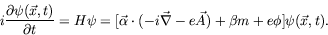(5.253)

Define the transformation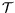such that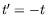and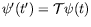, we have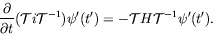(5.254)

Since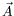is generated by currents which reverse sign when the sense of time is reversed,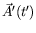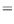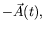(5.255)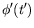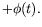(5.256)

Also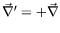, since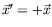. The transformation must cause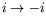to get the correct form, thereforecan be defined as:

1. take complex conjugate,
2. multiply by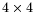constant matrix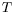.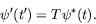(5.257)

Therefore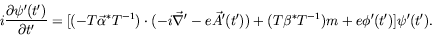(5.258)

This impliesmust commute with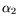and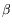and anticommute with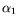and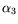. Therefore we can try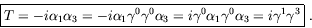(5.259)

The phase factor is arbitrary.

We applyto a plane-wave solution for a free particle of positive energy. Since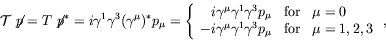(5.260)

and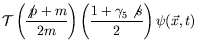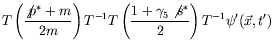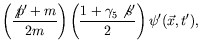(5.261)

where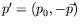;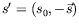Thereforeprojects out a free-particle solution with reversed direction of momentum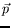and spin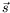. This is known as Wigner time reversal''.Next: Combined PCT Symmetry Up: Dirac Equation Previous: Charge Conjugation
Douglas M. Gingrich (gingrich@ ualberta.ca)
2004-03-18